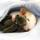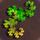## General Question# How do you do an optimal binary search on a two variable function?

Asked by LostInParadise (26818) March 30th, 2012

Suppose we have a symmetric strictly increasing function f(x,y), where x and y are non-negative integers. For a given value k, how do you go about finding all (x,y) such f(x,y) = k? Assume that we start knowing that f(0,y0) is an upper bound.

I was working with this and found that it was not as easy as it seems. Suppose that we find that f(3,7) < k < f(3,8). When we look at f(7,y), because of the symmetry and increasing properties of the function, we know that f(7,3) < k < f(7,8).

Observing members: 0Composing members: 0You need to clarify your terminology a little bit. Symmetric about the diagonal? Strictly increasing with respect to which ordering of the pairs?

ratboy (15157)“Great Answer” (1) Flag as…What I meant by symmetric is f(x,y) = f(y,x), and by strictly increasing, I meant that for x’ >= x and y’ >= y, f(x’,y’) >= f(x,y) with equality only if x’=x and y’ =y

LostInParadise (26818)“Great Answer” (0) Flag as…Is there is prescribed relation between f(x,y) and f(x’,y’) when, say, x > x’ and y = y’ ?

ratboy (15157)“Great Answer” (0) Flag as…What I am thinking of is something like f(x,y) = x^3 + y^3. The idea is that f(x,y) increases if either x or y increases.

LostInParadise (26818)“Great Answer” (0) Flag as…Is k guaranteed to be an integer?

Also, am I interpreting your question correctly that it is known in advance some number m such that f(0,m) > k?

PhiNotPi (12677)“Great Answer” (0) Flag as…or